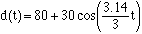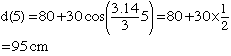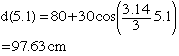Subject: pendulum problem MEKCA Calculus Student A pendulum hangs from the ceiling. as the pendulum swings, its distance,d cm, form one wall of the room depends on the number of seconds,t, since it was set in motion. assume that the equation for d as a function of t is: d=80+30cos3.14/3t, t>0. estimate the instantaneous rate of change of d at t=5 by finding the average rates for t=5 to 5.1, t=5 to 5.01, and t=5 to 5.001. Hi Mekca, The distance of the pendulum from the wall at time t seconds after it is set in motion isHence 5 seconds after it is set in motion (t = 5) its distance from the wall isAt time 5.1 seconds the pendulum distance from the wall isThus in the time from 5 to 5.1 seconds the distance from the wall has changed from 95 to 97.63 cm, and hence its average velocity was (97.63 -95)/(5.1 - 5) = 2.63/0.1 = 26.3 cm/sec. Now do the same for the time periods from 5 to 5.01 sec and from 5 to 5.001 sec. Cheers, harley Go to Math Central